# pair_style table discrepancies

Dear Lammps users,

I explored the pair_style table on a very simple system to familiarize myself with that style but ran into some discrepancies I would appreciate help with. My system is made of 2 atoms. One is fixed, the other is being pushed away by a non-bonded harmonic potential that I defined using a table. My input script is as follows:

units si
dimension 2
boundary p p p
atom_style atomic
region box block -1 1 -1 1 -1 1
create_box 1 box
create_atoms 1 single 0.0 0.0 0.0 #Atom 1 fixed
create_atoms 1 single 1e-6 0.0 0.0 #Atom 2 at inner cutoff distance
mass 1 1.0
group atom2 id 2
pair_style table linear 10
pair_coeff 1 1 pot.tab HARM_TEST
pair_write 1 1 10 rsq 1e-6 1e-3 tabledebug.txt PAIR_DEBUG
atom_modify sort 0 0.0 # no sorting
fix push atom2 nve
dump coor atom2 custom 100 dump.coor.lammpstrj id x fx
timestep 2e-5
run 10000

The pot.tab table file uses 10 points with RSQ spacing and harmonic parameters K=1.0, r0=1e-3 with inner and outer cutoff respectively 1e-6 and 1e-3. The table file is as follows:

# LAMMPS test table file harmonic potential

HARM_TEST
N 10 RSQ 1.000000e-06 1.000000e-03

1 1.000000e-06 9.980010e-07 1.998000e-03
2 3.333347e-04 4.444427e-07 1.333331e-03
3 4.714053e-04 2.794123e-07 1.057189e-03
4 5.773508e-04 1.786323e-07 8.452983e-04
5 6.666671e-04 1.111108e-07 6.666658e-04
6 7.453563e-04 6.484342e-08 5.092874e-04
7 8.164968e-04 3.367343e-08 3.670064e-04
8 8.819172e-04 1.394354e-08 2.361655e-04
9 9.428091e-04 3.270799e-09 1.143818e-04
10 1.000000e-03 0.000000e+00 0.000000e+00

The file tabledebug.txt obtained by the pair_write command is identical to the pot.tab table file used as an input. The tabledebug.txt file is as follows:

PAIR_DEBUG
N 10 RSQ 1e-006 0.001

1 1e-006 9.98001e-007 0.001998
2 0.000333334666664 4.444427e-007 0.00133333100000011
3 0.000471405345748221 2.794123e-007 0.001057189
4 0.000577350846539606 1.786323e-007 0.0008452983
5 0.000666667083333203 1.111108e-007 0.0006666658
6 0.000745356290642267 6.484342e-008 0.0005092874
7 0.000816496785051846 3.367343e-008 0.0003670064
8 0.000881917229676346 1.394354e-008 0.0002361655
9 0.000942809100507627 3.27079900000001e-009 0.0001143818
10 0.001 0 0

However, the position and the force on the atom2 obtained by the dump command are not consistent with the tabulated values. Especially, at low r, the force are highly overestimated. Selected lines of the dump file (first column = x, second column = fx) is as follows:

x fx

1.000000e-06 1.998000e-03

1.910920e-04 2.565790e-01

7.454560e-04 5.091220e-04

As we can see the force initially increases from r= 1.000000e-06 to r= 1.910920e-04 while the force should be strictly decreasing. The force finally decreases back to expected values.

I understand the problems of fitting splines to steep potentials and interpolating over these splines as discussed here: (https://lammps.sandia.gov/threads/msg40459.html https://lammps.sandia.gov/threads/msg19967.html). I tried varying the number of points and the spacing without success.

However in my case, I use a finite quadratic potential that does not diverge when r=0. What is more, according to the documentation, using N = Nfile = Ntable and RSQ spacing the interpolation table should “match exactly what is in the tabulated file (with effectively no preliminary interpolation)”. I was therefore expecting that the values of force would be interpolated linearly between the tabulated values but that is not the case.

I would be most grateful if you have any explanation that can explain this phenomenon.

Best,
Jibril Coulibaly

Have you used pair_write to output 1000s of values to see what the

energy/forces are for lots of points in between your 10 tabulated values?

That will tell you if you tabulated potential is good or bad.

Steve

Thank you Shafat and Steve for the prompt answers,

(1) I have output the potential with pair_write 10,000. The results are matching the tabulated data at the tabulated points. However the energy and force between these points are not linear, they are curved upwards. The amplitude of these curves decreases with increasing r. Between my first two tabulated values, the peak of the curve is very large compared to my input data. The curves are rather flat for distances beyond my second tabulated value (r=3.333347e-04). I am attaching pair_write files as well as figures of potential energy and force for clarity.
(2) I also increased the number of points as suggested. Increasing Nfile in my tabulated file to 100 (and increasing Ntable in my input file to 100 accordingly, the same behavior occurs, again, mostly between the first couple r values while smoothed out for larger r values.

The documentation of the pair_style table mentions that we must do the following to directly interpolate the tabulated values without pre-interpolation by cubic splines :

** The parameter “N” is required and its value is the number of table entries that follow. Note that this may be different than the N specified in the pair_style table command. Let Ntable = N in the pair_style command, and Nfile = “N” in the tabulated file. What LAMMPS does is a preliminary interpolation by creating splines using the Nfile tabulated values as nodal points. It uses these to interpolate energy and force values at Ntable different points. The resulting tables of length Ntable are then used as described above, when computing energy and force for individual pair distances. This means that if you want the interpolation tables of length Ntable to match exactly what is in the tabulated file (with effectively no preliminary interpolation), you should set Ntable = Nfile, and use the “RSQ” or “BITMAP” parameter. This is because the internal table abscissa is always RSQ (separation distance squared), for efficient lookup. **

To my understanding, I have been following these suggestions consistently but still end up with interpolated splines (the curves I observe) in my results instead of linear interpolation between tabulated values as I would have expected. I would appreciate help in identifying where my example/implementation breaks down and how I could obtain the desired behavior, i.e. a linear interpolation of energy and force between the tabulated values.

Thank you again,
Jibril Coulibaly

tabledebug_input10.txt (676 KB)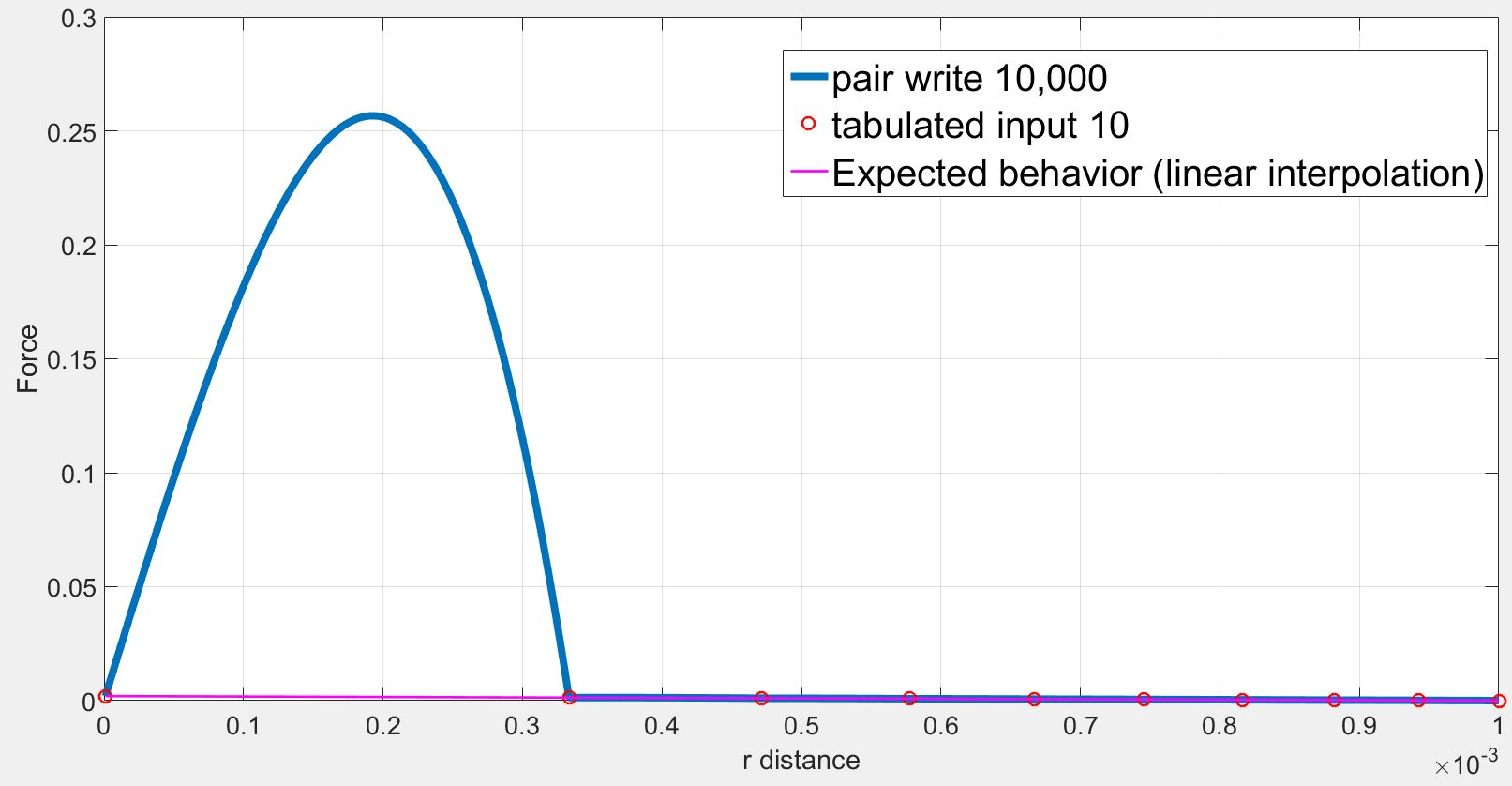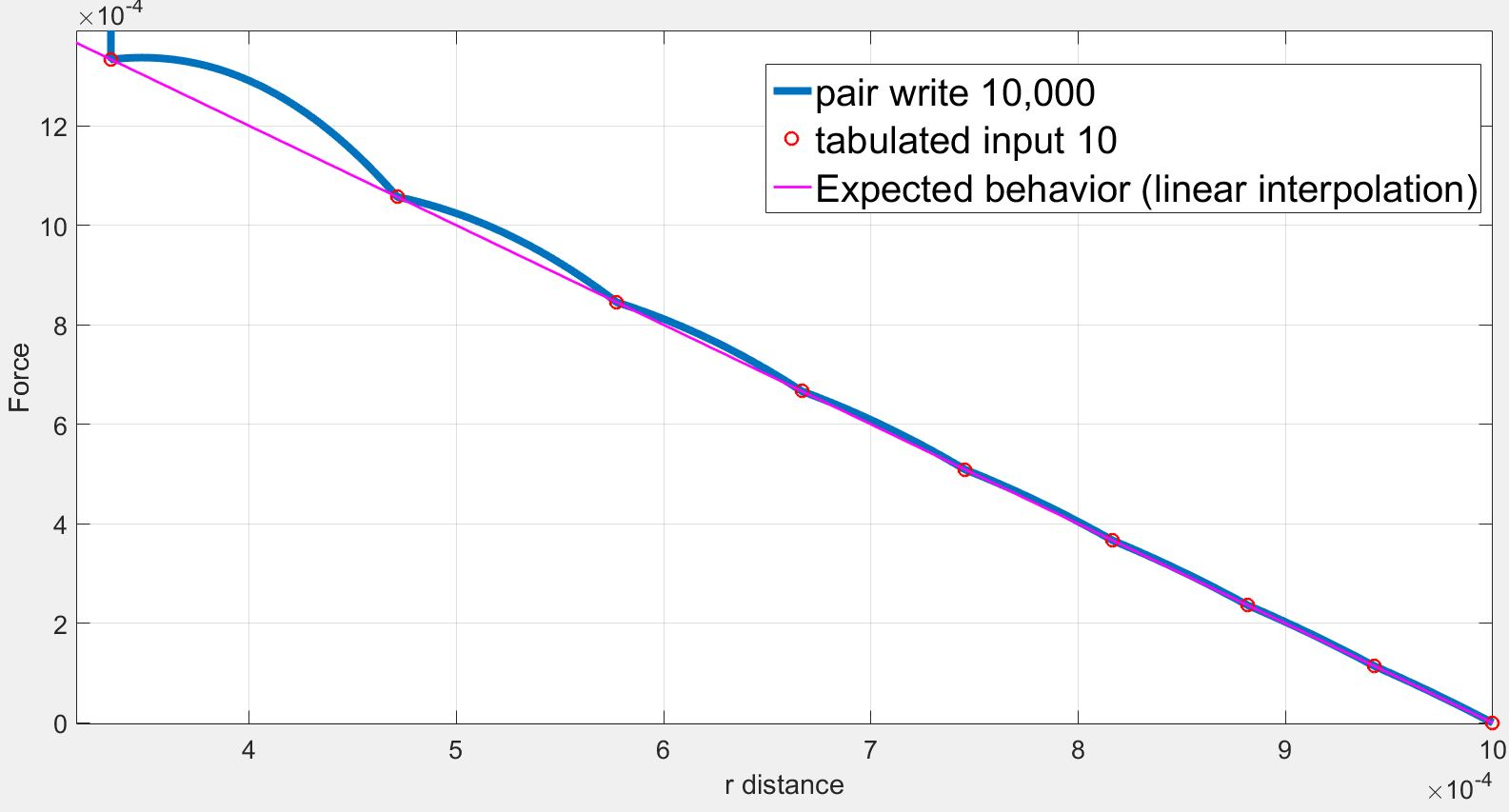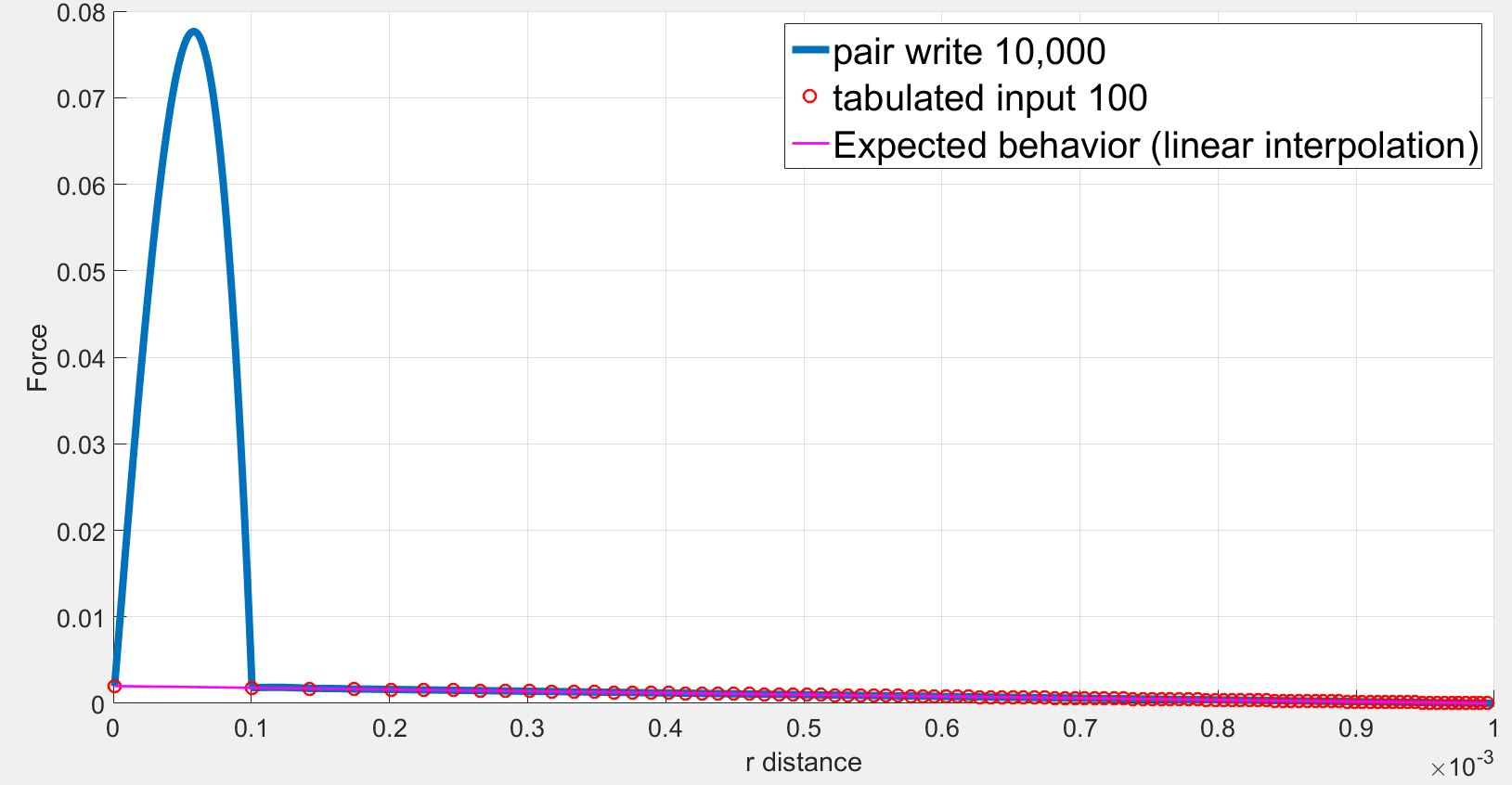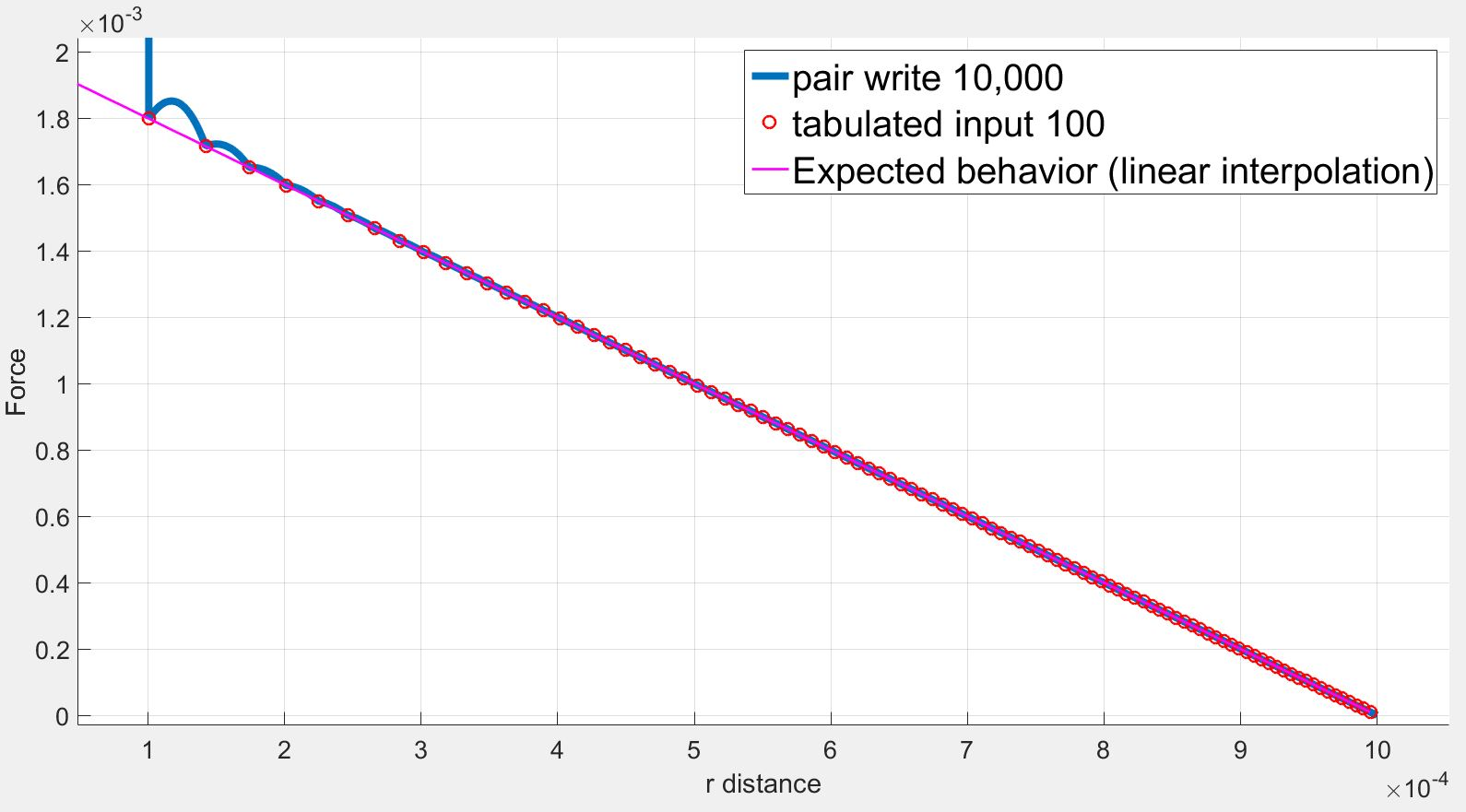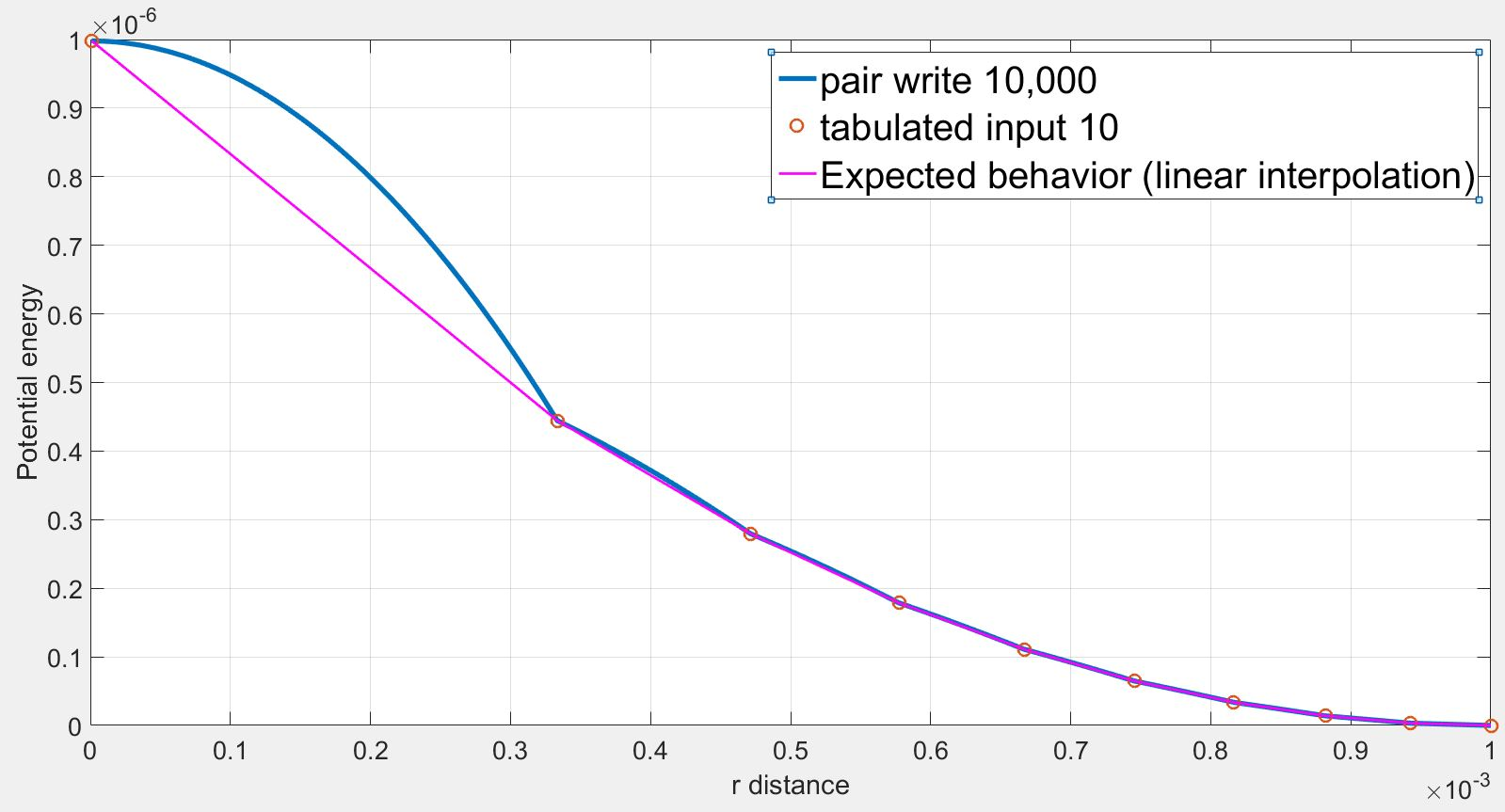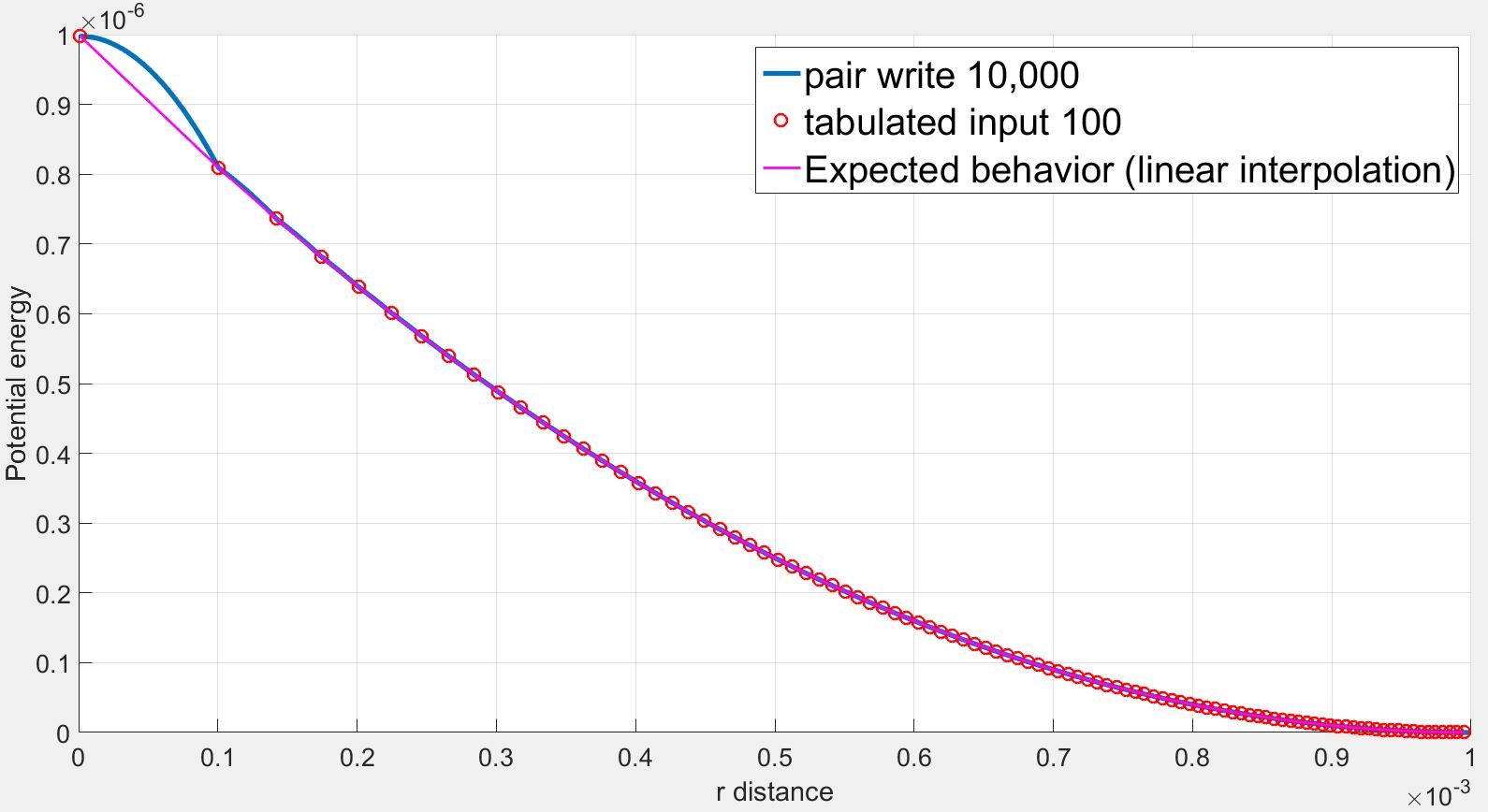tabledebug_input100.txt (677 KB)

I would try using many more than 10 initial points to define

the curve you want to fit to. If you’re having trouble

at one end it may also be that you need to define

the slope at that end correctly in order for the first

spline not to get confused.

Steve